# Texas Go Math Grade 3 Lesson 17.1 Answer Key Model Perimeter

Refer to our Texas Go Math Grade 3 Answer Key Pdf to score good marks in the exams. Test yourself by practicing the problems from Texas Go Math Grade 3 Lesson 17.1 Answer Key Model Perimeter.

## Texas Go Math Grade 3 Lesson 17.1 Answer Key Model Perimeter

Investigate

Perimeter is the distance around a figure.
Materials; geoboard, rubber bands

You can find the perimeter of a rectangle on a geoboard or on dot paper by counting the number of units on each side.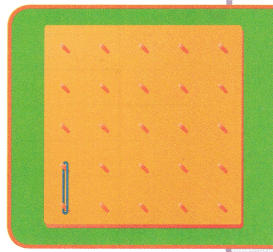A. Make a rectangle on the geoboard that is 3 units on two sides and 2 units on the other two sides.B. Draw your rectangle on the dot paper below.C. Write the length next to each side of your rectangle.D. Add the number of units on each side.
________ + ________ + ________ + _________ = __________
3+2+3+2=10

E. So, the perimeter of the rectangle is __________ units.
So, perimeter of the rectangle is 10 units.

How would the perimeter of the rectangle change if the length of two of the sides was 4 units instead of 3 units?
The perimeter would be 12 units if the length is 4 units instead of 3 units.

Make Connections

You can also use grid paper to find the perimeter of figures by counting the number of units on each side.

Start at the arrow and trace the perimeter. Begin counting with 1. Continue counting each unit around the figure until you have counted each unit.Explanation:
Perimeter of shape A is 16 units and Perimeter of shape B is 14 units.

Math Talk
Mathematical Processes

If a rectangle has a perimeter of 12 units, how many units wide and how many units long could it be? Explain.
The length of the rectangle cound be 2 units wide and 4 units long.

Share and Show

Find the perimeter of the figure. Each unit is 1 centimeter.

Question 1.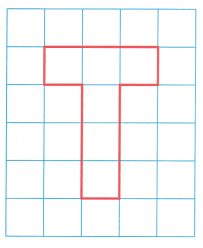_____________ centimetersExplanation:
The perimeter of the given figure is 12 centimeters.

Question 2._____________ centimeters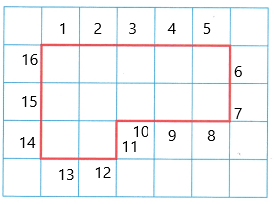Explanation:
The perimeter of the given figure is 16 centimeters.

Problem Solving

H.O.T. Multi-Step What’s the Error?

Question 3.
Kevin is solving perimeter problems. He counts the units and says that the perimeter of this figure is 18 units.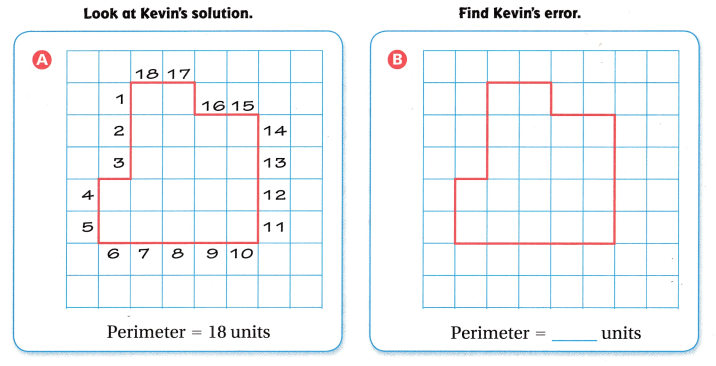Use the grid on the right to find the correct perimeter of the figure above.Question 4.
Apply Describe the error Kevin made.
Kevin wrongly counted the sides of the figure given.

Question 5.
Use Diagrams Circle the places in the drawing of Kevin’s solution where he made an error.
I circled the places besides 3 and 16 as Kevin made the errors there.

Question 6.
H.O.T. Write Math Explain how to find the length of each side of a triangle with sides of equal length, and a perimeter of 27 inches.
The perimeter of a triangle is 3 times of itd side.Perimeter is 27inches then to find the side of the triangle divide the perimeter by 3
27 divided by 3 is 9
Therefore, length of each side of a triangle with sides of equal length is 9.

Question 7.
Joseph drew a game court on grid paper. Each unit is 1 meter. What is the perimeter of the game court?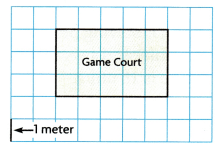(A) 18 meters
(B) 15 meters
(C) 16 meters
(D) 8 meters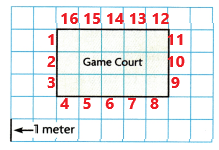Explanation:
The perimeter of the game court drew by Joseph is Option (C)16 meters.

Question 8.
Val is designing a dog park. She needs to find the length for a fence around the park. Each unit is 1 yard. What is the perimeter of the park?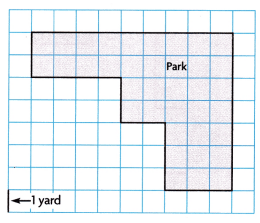(A) 29 yards
(B) 32 yards
(C) 47 yards
(D) 34 yards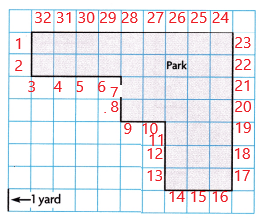Explanation:
The perimeter of dog park designed by Val is Option (B) 32 yards.

Question 9.
Multi-Step Greg is framing two rectangular paintings. One painting has side lengths of 3 inches and 9 inches. The other painting has side lengths of 4 inches and 6 inches. How many inches of framing material will Greg need for the two paintings?
(A) 48 inches
(B) 51 inches
(C) 22 inches
(D) 44 inches
D. 44 inches of framing material greg will need for the two paintings.

Explanation:
Greg is framing two rectangular paintings. One painting has side lengths of 3 inches and 9 inches.
perimeter of a rectangle is 2(l+w)
2(9+3) = 2(11) = 22 inches
The other painting has side lengths of 4 inches and 6 inches
perimeter of a rectangle is 2(l+w)
2(4+6) = 2(10) = 20 inches
The sum of perimeters of both painting is 22+20 = 44 inches.
Therefore, 44 inches of framing material greg will need for the two paintings.

Texas Test Prep

Question 10.
Naomi drew this design for a quilt block. What is the perimeter of the design?(A) 12 units
(B) 16 units
(C) 14 units
(D) 18 unitsExplanation:
Naomi drew this design for a quilt block. The perimeter of the design is 16 units.

### Texas Go Math Grade 3 Lesson 17.1 Homework and Practice Answer Key

Find the perimeter of the figure. Each unit is 1 centimeter.

Question 1.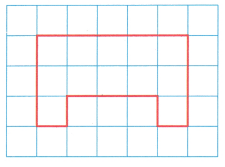__________ centimeters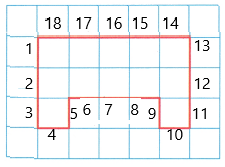Explanation:
The perimeter of the above figure is 18 units that is 18 centimeters.

Question 2.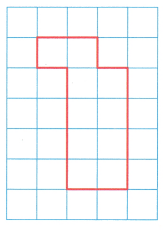__________ centimeters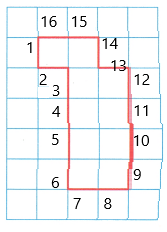Explanation:
The perimeter of the above figure is 16 units that is 16 centimeters.

Problem Solving

Question 3.
Alyssa says the perimeter of this figure is 13 units. Describe the error Alyssa made.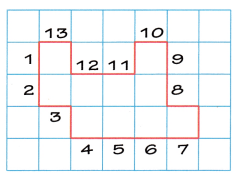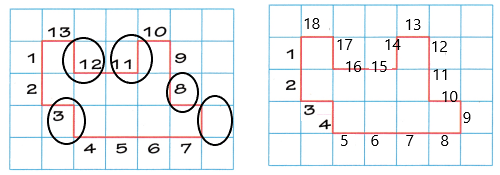Explanation:
Alyssa says the perimeter of this figure is 13 units but she made errorsin counting the sides of the figure.I circled the places of errors made by Alyssa.
The perimeter of the given figure is 18 units.

Question 4.
Draw your own figure on the grid. Find the perimeter.Perimeter = __________ units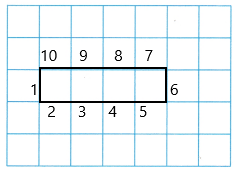Explanation:
I drew a rectangle of my own.The length is 4units and width is 1 unit.I marked the units to find the perimeter.
Perimeter of the rectangle i drew is 10units.

Lesson Check

Question 5.
Paula drew this plan for a new backyard shed. Each unit is 1 foot. What is the perimeter of the shed?(A) 24 feet
(B) 20 feet
(C) 18 feet
(D) 42 feet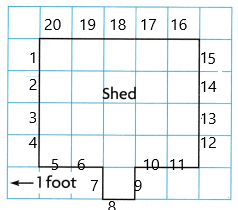Explanation:
Paula drew this plan for a new backyard shed
Perimteter of the shed is 20units.
As 1 unit is equal to 1 foot. So, perimeter of the shed is 20feet.

Question 6.
Andy drew this plan for a fence in his yard. Each unit is 1 meter. What is the perimeter of the fence?(A) 18 meter
(B) 24 meter
(C) 22 meter
(D) 36 meter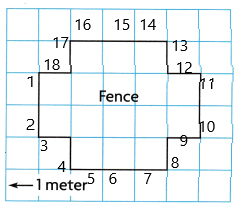Explanation:
Andy drew this plan for a fence in his yard.
Perimeter of the yard is 18 units
As 1 unit is equal to 1 meter, the perimeter of the yard is 18 meters.

Question 7.
Multi-Step Latoya sews a border around two quilts. One quilt has side lengths of 4 feet and 6 feet. Another quilt has side lengths of 8 feet and 6 feet. How many feet of border does she sew?
(A) 28 feet
(B) 20 feet
(C) 48 feet
(D) 42 feet
C. 48 feet of border Latoya sews round two quilts

Explanation:
Latoya sews a border around two quilts. One quilt has side lengths of 4 feet and 6 feet.
perimeter of a rectangle is 2(l+w)
2(4+6) = 2(10) = 20 inches
Another quilt has side lengths of 8 feet and 6 feet.
perimeter of a rectangle is 2(l+w)
2(8+6) = 2(14) = 28 inches
The sum of perimeters of both quilts is 20+28 = 44 feet.
Therefore, 48 feet of border Latoya sews round two quilts.

Question 8.
Multi-Step Roland buys baseboards for two rectangular rooms. One room has side lengths of 5 meters and 7 meters. The second room has side lengths of 6 meters and 9 meters. How many meters of baseboard does Roland buy?
(A) 50 meters
(B) 30 meters
(C) 24 meters
(D) 54 meters